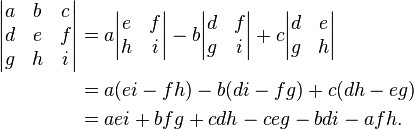# Minors and Co-factors

To evaluate the determinant of order 3 or above, you need to define minors and co-factors.

### Minors

Let |A| = |[aij]| be a determinant of order n. The minor of an arbitrary element aij is the determinant obtained by deleting the ith row and jth column in which the element aij stands. The minor of aij is denoted by Mij.

### Co-factors

The co-factor is a signed minor. The co-factor of aij is denoted by Aij and is defined as

Aij = (−1)i+j Mij

### Determinant Expansion